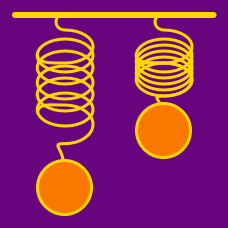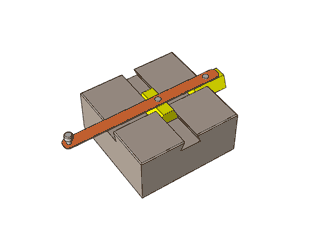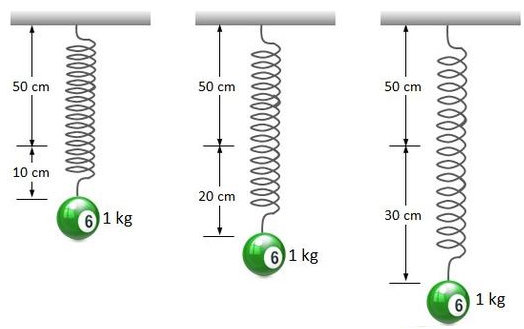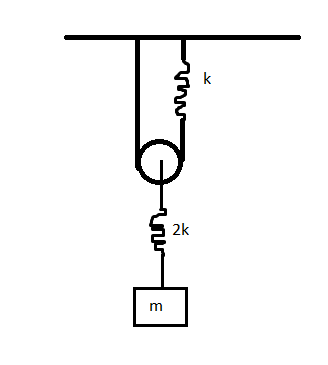Classical Mechanics

# Simple Harmonic Motion: Level 2-3 ChallengesIn the figure two blocks are executing simple harmonic motion of same frequency in such a way that when one is at position of maximum displacement then other is at mean position. Now what is the path of external end point of rod.When a ball with mass $1\text{ kg}$ is hung on a $50\text{ cm}$ long spring, the length of the spring is increased by $10\text{ cm}$ in steady state, as shown in the far left diagram. Now, suppose that we pull this ball further down and let it go. Then it will show simple harmonic oscillations. What will be the periods of these oscillations when we pull down the ball by $10$ cm and $20$ cm, respectively, as shown in the middle and right diagrams above?

Gravitational acceleration is $10 \text{ m/s}^2$.

What is the ratio of the period of a pendulum on Mars to the period of the same pendulum on Earth?

Details and assumptions

Gravity on Earth is $9.8~m/s^{2}$

Gravity on Mars is $3.71~m/s^{2}$If the time period of simple harmonic motion of the rectangular block can be represented as $T=2\pi \sqrt{\frac{am}{bk}}$ for coprime positive integers $a,b$.

Find $\sqrt{a^2+b^2}$ up to two decimal places.

Details and Assumptions

• Pulley is smooth and massless, and the strings are light and inextensible.
• Gravity is present.
• The crooked lines in the figure represents springs of spring constant $k$ and $2k$ as shown.

-Take the amplitude of oscillations to be very small.

A particle oscillating in simple harmonic motion has its motion timed at $t = 0 s$ when it is at the $50 cm$ mark. It travels between the $50 cm$ and $70 cm$ marks with a period of $2.0 s$. Where is the position of the particle at time $t = 0.75 s$ ?

×